# 2.2: Use a General Strategy to Solve Linear Equations

•• OpenStax
• OpenStax
$$\newcommand{\vecs}{\overset { \rightharpoonup} {\mathbf{#1}} }$$ $$\newcommand{\vecd}{\overset{-\!-\!\rightharpoonup}{\vphantom{a}\smash {#1}}}$$$$\newcommand{\id}{\mathrm{id}}$$ $$\newcommand{\Span}{\mathrm{span}}$$ $$\newcommand{\kernel}{\mathrm{null}\,}$$ $$\newcommand{\range}{\mathrm{range}\,}$$ $$\newcommand{\RealPart}{\mathrm{Re}}$$ $$\newcommand{\ImaginaryPart}{\mathrm{Im}}$$ $$\newcommand{\Argument}{\mathrm{Arg}}$$ $$\newcommand{\norm}{\| #1 \|}$$ $$\newcommand{\inner}{\langle #1, #2 \rangle}$$ $$\newcommand{\Span}{\mathrm{span}}$$ $$\newcommand{\id}{\mathrm{id}}$$ $$\newcommand{\Span}{\mathrm{span}}$$ $$\newcommand{\kernel}{\mathrm{null}\,}$$ $$\newcommand{\range}{\mathrm{range}\,}$$ $$\newcommand{\RealPart}{\mathrm{Re}}$$ $$\newcommand{\ImaginaryPart}{\mathrm{Im}}$$ $$\newcommand{\Argument}{\mathrm{Arg}}$$ $$\newcommand{\norm}{\| #1 \|}$$ $$\newcommand{\inner}{\langle #1, #2 \rangle}$$ $$\newcommand{\Span}{\mathrm{span}}$$$$\newcommand{\AA}{\unicode[.8,0]{x212B}}$$

##### Summary

By the end of this section, you will be able to:

• Use the commutative and associative properties
• Use the properties of identity, inverse, and zero
• Simplify expressions using the Distributive Property

Before you get started, take this readiness quiz.

1. Simplify: $$\frac{3}{2}(12x+20)$$.
2. Simplify: $$5−2(n+1)$$.
3. Find the LCD of $$\frac{5}{6}$$ and $$\frac{1}{4}$$.

## Solve Linear Equations Using a General Strategy

Solving an equation is like discovering the answer to a puzzle. The purpose in solving an equation is to find the value or values of the variable that makes it a true statement. Any value of the variable that makes the equation true is called a solution to the equation. It is the answer to the puzzle!

##### Solution OF AN EQUATION

A solution of an equation is a value of a variable that makes a true statement when substituted into the equation.

To determine whether a number is a solution to an equation, we substitute the value for the variable in the equation. If the resulting equation is a true statement, then the number is a solution of the equation.

##### DETERMINE WHETHER A NUMBER IS A Solution TO AN EQUATION
1. Substitute the number for the variable in the equation.
2. Simplify the expressions on both sides of the equation.
3. Determine whether the resulting equation is true.
• If it is true, the number is a solution.
• If it is not true, the number is not a solution.
##### Example $$\PageIndex{1}$$

Determine whether the values are solutions to the equation: $$5y+3=10y−4$$.

1. $$y=\frac{3}{5}$$
2. $$y=\frac{7}{5}$$
Solution

Since a solution to an equation is a value of the variable that makes the equation true, begin by substituting the value of the solution for the variable.

a.

 $$5 y+3=10 y-4$$ Substitute $$\color{rec}\frac{3}{5}$$ for $$y$$ $$5\left( \color{red} \frac{3}{5} \color{black}\right)+3 \stackrel{?}{=} 10\left( \color{red}\frac{3}{5} \color{black}\right)-4$$ Multiply. $$3+3\stackrel{?}{=} 6-4$$ Simplify. $$6 \neq 2$$

Since $$y=\frac{3}{5}$$ does not result in a true equation, $$y=\frac{3}{5}$$ is not a solution to the equation $$5y+3=10y−4.$$

b.

 $$5 y+3=10 y-4$$ Substitute $$\color{red} \frac{7}{5}$$ for $$y$$ $$5\left(\color{red} \frac{7}{5} \color{black}\right)+3 \stackrel{?}{=} 10\left(\color{red}\frac{7}{5}\color{back}\right)-4$$ Multiply. $$7+3 \stackrel{?}{=} 14-4$$ Simplify. $$10=10 \checkmark$$

Since $$y=\frac{7}{5}$$ results in a true equation, $$y=\frac{7}{5}$$ is a solution to the equation $$5y+3=10y−4.$$

##### Exercise $$\PageIndex{1A}$$

Determine whether the values are solutions to the equation: $$9y+2=6y+3.$$

1. $$y=\frac{4}{3}$$
2. $$y=\frac{1}{3}$$

no

yes

##### Exercise $$\PageIndex{1B}$$

Determine whether the values are solutions to the equation: $$4x−2=2x+1$$.

1. $$x=\frac{3}{2}$$
2. $$x=−\frac{1}{2}$$

yes

no

There are many types of equations that we will learn to solve. In this section we will focus on a linear equation.

##### LINEAR EQUATION

A linear equation is an equation in one variable that can be written, where $$a$$ and $$b$$ are real numbers and $$a≠0$$, as:

$ax+b=0$

To solve a linear equation it is a good idea to have an overall strategy that can be used to solve any linear equation. In the next example, we will give the steps of a general strategy for solving any linear equation. Simplifying each side of the equation as much as possible first makes the rest of the steps easier.

##### EXAMPLE $$\PageIndex{2}$$

Solve: $$7(n−3)−8=−15$$Solve: $$2(m−4)+3=−1.$$

$$m=2$$

##### Exercise $$\PageIndex{2B}$$

Solve: $$5(a−3)+5=−10.$$

$$a=0$$

These steps are summarized in the General Strategy for Solving Linear Equations below.

##### SOLVE LINEAR EQUATIONS USING A GENERAL STRATEGY
1. Simplify each side of the equation as much as possible.
Use the Distributive Property to remove any parentheses.
Combine like terms.
2. Collect all the variable terms on one side of the equation.

Use the Addition or Subtraction Property of Equality.

3. Collect all the constant terms on the other side of the equation.

Use the Addition or Subtraction Property of Equality.

4. Make the coefficient of the variable term equal to 1.

Use the Multiplication or Division Property of Equality.

State the solution to the equation.

5. Check the solution.

Substitute the solution into the original equation to make sure the result is a true statement.

##### EXAMPLE $$\PageIndex{3}$$

Solve: $$\frac{2}{3}(3m−6)=5−m$$.

 $$\frac{2}{3}(3 m-6)=5-m$$ Distribute. $$2 m-4=5-m$$ Add $$m$$ to both sides to get the variables only on the left.Simplify. $$3 m-4=5$$ Add $$4$$ to both sides to get constants only on the right.Simplify. $$3 m=9$$ Divide both sides by three.Simplify. $$m=3$$
 Check:Let $$m=3$$.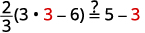##### Exercise $$\PageIndex{3A}$$

Solve: $$\frac{1}{3}(6u+3)=7−u$$.

$$u=2$$

##### Exercise $$\PageIndex{3B}$$

Solve: $$\frac{2}{3}(9x−12)=8+2x$$.

$$x=4$$

We can solve equations by getting all the variable terms to either side of the equal sign. By collecting the variable terms on the side where the coefficient of the variable is larger, we avoid working with some negatives. This will be a good strategy when we solve inequalities later in this chapter. It also helps us prevent errors with negatives.

##### EXAMPLE $$\PageIndex{4}$$

Solve: $$4(x−1)−2=5(2x+3)+6$$.

 $$4(x-1)-2=5(2 x+3)+6$$ Distribute. $$4 x-4-2=10 x+15+6$$ Combine like terms. $$4 x-6=10 x+21$$ Subtract $$4x$$ from each side to get the variables only on the right since $$10>4$$. $$4 x \color{red} -4 \color{black} x-6=10 x \color{red}-4 x \color{black}+21$$ Simplify. $$-6=6 x+21$$ Subtract $$21$$ from each side to get the constants on left. $$-6 \color{red} -21 \color{black} =6 x+21 \color{red}-21$$ Simplify. $$-27=6 x$$ Divide both sides by $$6$$. $$\frac{-27}{\color{red}6} \color{black}=\frac{6 x}{\color{red}6}$$ Simplify. $$-\frac{9}{2}=x$$ Check: $$4(x-1)-2=5(2 x+3)+6$$\) Let $$x=−92$$.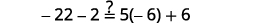##### Exercise $$\PageIndex{4A}$$

Solve: $$6(p−3)−7=5(4p+3)−12.$$

$$p=−2$$

##### Exercise $$\PageIndex{4B}$$

Solve: $$8(q+1)−5=3(2q−4)−1.$$

$$q=−8$$

##### EXAMPLE $$\PageIndex{5}$$

Solve: $$10[3−8(2s−5)]=15(40−5s)$$.

 $$10[3-8(2 s-5)]=15(40-5 s)$$ Simplify from the innermost parentheses first. $$10[3-16 s+40]=15(40-5 s)$$ Combine like terms in the brackets. $$10[43-16 s]=15(40-5 s)$$ Distribute. $$430-160 s=600-75 s$$ Add $$160s$$ to both sides to get the $$160s$$ to both sides to get the variables to the right.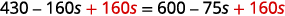Simplify. $$430=600+85 s$$ Subtract $$600$$ from both sides to get the constants to the left.Simplify. $$-170=85 s$$ Divide both sides by $$85$$.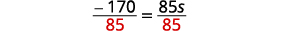Simplify. $$-2=s,$$ so $$s = -2$$ Check: $$10[3-8(2 s-5)]=15(40-5 s)$$ Let $$s=−2$$.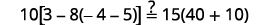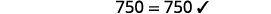##### Exercise $$\PageIndex{5A}$$

Solve: $$6[4−2(7y−1)]=8(13−8y)$$.

$$y=−\frac{17}{5}$$

##### Exercise $$\PageIndex{5B}$$

Solve: $$12[1−5(4z−1)]=3(24+11z).$$

$$z=0$$

## Classify Equations

Whether or not an equation is true depends on the value of the variable. The equation $$7x+8=−13$$ is true when we replace the variable, x, with the value $$−3$$, but not true when we replace x with any other value. An equation like this is called a conditional equation. All the equations we have solved so far are conditional equations.

##### CONDITIONAL EQUATION

An equation that is true for one or more values of the variable and false for all other values of the variable is a conditional equation.

Now let’s consider the equation $$7y+14=7(y+2)$$. Do you recognize that the left side and the right side are equivalent? Let’s see what happens when we solve for y.

Solve:

 $$7 y+14=7(y+2)$$ Distribute. $$7 y+14=7 y+14$$ Subtract $$7y$$ to each side to get the $$y’$$s to one side. $$7 y \color{red}-7 y \color{black} +14=7 y \color{red} -7 y \color{black}+14$$ Simplify—the $$y$$'s are eliminated. $$14=14$$ But $$14=14$$ is true.

This means that the equation $$7y+14=7(y+2)$$ is true for any value of $$y$$. We say the solution to the equation is all of the real numbers. An equation that is true for any value of the variable is called an identity.

##### IDENTITY

An equation that is true for any value of the variable is called an identity.

The solution of an identity is valid for all real numbers.

What happens when we solve the equation $$−8z=−8z+9?$$

Solve:

 $$-8 z=-8 z+9$$ Add $$8z$$ to both sides to leave the constant alone on the right. $$-8 z \color{red} +8 z \color{black}=-8 z \color{red}+8 z \color{black} +9$$ Simplify—the $$z$$'s are eliminated. $$0 \neq 9$$ But $$0≠9$$.

Solving the equation $$−8z=−8z+9$$ led to the false statement $$0=9$$. The equation $$−8z=−8z+9$$ will not be true for any value of $$z$$. It has no solution. An equation that has no solution, or that is false for all values of the variable, is called a contradiction.

An equation that is false for all values of the variable is called a contradiction.

The next few examples will ask us to classify an equation as conditional, an identity, or as a contradiction.

##### EXAMPLE $$\PageIndex{6}$$

Classify the equation as a conditional equation, an identity, or a contradiction and then state the solution: $$6(2n−1)+3=2n−8+5(2n+1)$$.

 $$6(2 n-1)+3=2 n-8+5(2 n+1)$$ Distribute. $$12 n-6+3=2 n-8+10 n+5$$ Combine like terms. $$12 n-3=12 n-3$$ Subtract $$12n$$ from each side to get the $$n$$'s to one side.Simplify. $$-3=-3$$ This is a true statement. The equation is an identity. The solution is all real numbers.
##### Exercise $$\PageIndex{6A}$$

Classify the equation as a conditional equation, an identity, or a contradiction and then state the solution: $$4+9(3x−7)=−42x−13+23(3x−2).$$

identity; all real numbers

##### Exercise $$\PageIndex{6B}$$

Classify the equation as a conditional equation, an identity, or a contradiction and then state the solution: $$8(1−3x)+15(2x+7)=2(x+50)+4(x+3)+1.$$

identity; all real numbers

##### EXAMPLE $$\PageIndex{7}$$

Classify the equation as a conditional equation, an identity, or a contradiction and then state the solution: $$8+3(a−4)=0$$.

 $$8+3(a-4)=0$$ Distribute. $$8+3 a-12=0$$ Combine like terms. $$3 a-4=0$$ Add $$4$$ to both sides. $$3 a-4 \color{red}+4 \color{black}=0 \color{red}+4$$ Simplify. $$3 a=4$$ Divide. $$\frac{3 a}{\color{red}3} \color{black}=\frac{4}{\color{red}3}$$ Simplify. $$a=\frac{4}{3}$$ The equation is true when $$a=\frac{4}{3}$$. This is a conditional equation. The solution is $$a=\frac{4}{3}$$.
##### Exercise $$\PageIndex{7A}$$

Classify the equation as a conditional equation, an identity, or a contradiction and then state the solution: $$11(q+3)−5=19$$.

conditional equation; $$q=−\frac{9}{11}$$

##### Exercise $$\PageIndex{7B}$$

Classify the equation as a conditional equation, an identity, or a contradiction and then state the solution: $$6+14(k−8)=95$$.

conditional equation; $$k=\frac{201}{14}$$

##### EXAMPLE $$\PageIndex{8}$$

Classify the equation as a conditional equation, an identity, or a contradiction and then state the solution: $$5m+3(9+3m)=2(7m−11)$$.

 $$5 m+3(9+3 m)=2(7 m-11)$$ Distribute. $$5 m+27+9 m=14 m-22$$ Combine like terms. $$14 m+27=14 m-22$$ Subtract $$14m$$ from both sides. $$14 m+27 \color{red}-14 m \color{black}=14 m-22 \color{red}-14 m$$ Simplify. $$27 \neq-22$$ But $$27≠−22$$. The equation is a contradiction. It has no solution.

Classify the equation as a conditional equation, an identity, or a contradiction and then state the solution: $$12c+5(5+3c)=3(9c−4)$$.

##### Exercise $$\PageIndex{8B}$$

Classify the equation as a conditional equation, an identity, or a contradiction and then state the solution:$$4(7d+18)=13(3d−2)−11d$$.

We summarize the methods for classifying equations in the table.

Type of equation What happens when you solve it? Solution
Conditional Equation True for one or more values of the variables and false for all other values One or more values
Identity True for any value of the variable All real numbers
Contradiction False for all values of the variable No solution

## Solve Equations with Fraction or Decimal Coefficients

We could use the General Strategy to solve the next example. This method would work fine, but many students do not feel very confident when they see all those fractions. So, we are going to show an alternate method to solve equations with fractions. This alternate method eliminates the fractions.

We will apply the Multiplication Property of Equality and multiply both sides of an equation by the least common denominator (LCD) of all the fractions in the equation. The result of this operation will be a new equation, equivalent to the first, but without fractions. This process is called clearing the equation of fractions.

To clear an equation of decimals, we think of all the decimals in their fraction form and then find the LCD of those denominators.

##### Exercise $$\PageIndex{9A}$$

Solve: $$\frac{1}{12}x+\frac{5}{6}=\frac{3}{4}$$.##### Exercise $$\PageIndex{9B}$$

Solve: $$\frac{1}{4}x+\frac{1}{2}=\frac{5}{8}$$.

$$x=\frac{1}{2}$$

##### Exercise $$\PageIndex{9C}$$

Solve: $$\frac{1}{8}x+\frac{1}{2}=\frac{1}{4}$$.

$$x=−2$$

Notice in the previous example, once we cleared the equation of fractions, the equation was like those we solved earlier in this chapter. We changed the problem to one we already knew how to solve. We then used the General Strategy for Solving Linear Equations.

##### SOLVE EQUATIONS WITH FRACTION OR DECIMAL COEFFICIENTS.
1. Find the least common denominator (LCD) of all the fractions and decimals (in fraction form) in the equation.
2. Multiply both sides of the equation by that LCD. This clears the fractions and decimals.
3. Solve using the General Strategy for Solving Linear Equations.
##### EXAMPLE $$\PageIndex{10}$$

Solve: $$5=\frac{1}{2}y+\frac{2}{3}y−\frac{3}{4}y$$.

We want to clear the fractions by multiplying both sides of the equation by the LCD of all the fractions in the equation.

 Find the LCD of all fractions in the equation. $$5=\frac{1}{2} y+\frac{2}{3} y-\frac{3}{4} y$$ The LCD is $$12$$. Multiply both sides of the equation by $$12$$. $$\color{red}12 \color{black}(5)=\color{red}12 \color{black} \cdot\left(\frac{1}{2} y+\frac{2}{3} y-\frac{3}{4} y\right)$$ Distribute. $$12(5)=12 \cdot \frac{1}{2} y+12 \cdot \frac{2}{3} y-12 \cdot \frac{3}{4} y$$ Simplify—notice, no more fractions. $$60=6 y+8 y-9 y$$ Combine like terms. $$60=5 y$$ Divide by five. $$\frac{60}{\color{red}5} \color{black}=\frac{5 y}{\color{red}5}$$ Simplify. $$12=y$$ Check: $$5=\frac{1}{2} y+\frac{2}{3} y-\frac{3}{4} y$$ Let $$y=12$$.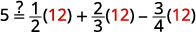##### Exercise $$\PageIndex{10A}$$

Solve: $$7=\frac{1}{2}x+\frac{3}{4}x−\frac{2}{3}x$$.

$$x=12$$

##### Exercise $$\PageIndex{10B}$$

Solve: $$−1=\frac{1}{2}u+\frac{1}{4}u−\frac{2}{3}u$$.

$$u=−12$$

In the next example, we’ll distribute before we clear the fractions.

##### EXAMPLE $$\PageIndex{11}$$

Solve: $$\frac{1}{2}(y−5)=\frac{1}{4}(y−1)$$.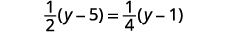Distribute.Simplify.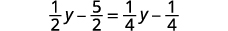Multiply by the LCD, four.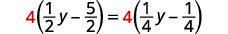Distribute.Simplify.Collect the variables to the left.Simplify.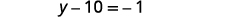Collect the constants to the right.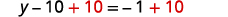Simplify.An alternate way to solve this equation is to clear the fractions without distributing first. If you multiply the factors correctly, this method will be easier.Multiply by the LCD, $$4$$.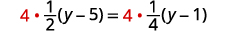Multiply four times the fractions.Distribute.Collect the variables to the left.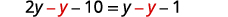Simplify.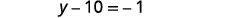Collect the constants to the right.Simplify.Check: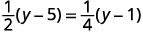Let $$y=9$$.Finish the check on your own.
##### Exercise $$\PageIndex{11A}$$

Solve: $$\frac{1}{5}(n+3)=\frac{1}{4}(n+2)$$.

$$n=2$$

##### Exercise $$\PageIndex{11B}$$

Solve: $$\frac{1}{2}(m−3)=\frac{1}{4}(m−7)$$.

$$m=−1$$

When you multiply both sides of an equation by the LCD of the fractions, make sure you multiply each term by the LCD—even if it does not contain a fraction.

##### EXAMPLE $$\PageIndex{12}$$

Solve: $$\frac{4q+3}{2}+6=\frac{3q+5}{4}$$

 $$\frac{4 q+3}{2}+6=\frac{3 q+5}{4}$$ Multiply both sides by the LCD, $$4$$.Distribute.Simplify. $$2(4 q+3)+24=3 q+5$$ $$8 q+6+24=3 q+5$$ $$8 q+30=3 q+5$$ Collect the variables to the left.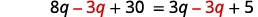Simplify. $$5 q+30=5$$ Collect the constants to the right.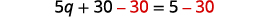Simplify. $$5 q=-25$$ Divide both sides by five.Simplify. $$q=-5$$ Check: $$\frac{4 q+3}{2}+6=\frac{3 q+5}{4}$$ Let $$q=−5.$$Finish the check on your own.

Solve: $$\frac{3r+5}{6}+1=\frac{4r+3}{3}$$.

$$r=3$$

##### Exercise $$\PageIndex{12B}$$

Solve: $$\frac{2s+3}{2}+1=\frac{3s+2}{4}$$.

$$s=−8$$

Some equations have decimals in them. This kind of equation may occur when we solve problems dealing with money or percentages. But decimals can also be expressed as fractions. For example, $$0.7=\frac{7}{10}$$ and $$0.29=\frac{29}{100}$$. So, with an equation with decimals, we can use the same method we used to clear fractions—multiply both sides of the equation by the least common denominator.

The next example uses an equation that is typical of the ones we will see in the money applications in a later section. Notice that we will clear all decimals by multiplying by the LCD of their fraction form.

Solve: $$0.25x+0.05(x+3)=2.85$$.

Look at the decimals and think of the equivalent fractions:

$0.25=\frac{25}{100}, \; \; \; \;\;\;\;\; 0.05=\frac{5}{100}, \;\;\;\;\;\;\;\; 2.85=2\frac{85}{100}.$

Notice, the LCD is $$100$$. By multiplying by the LCD we will clear the decimals from the equation.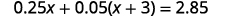Distribute first.Combine like terms.To clear decimals, multiply by $$100$$.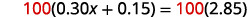Distribute.Subtract $$15$$ from both sides.Simplify.Divide by $$30$$.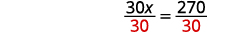Simplify.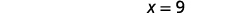Check it yourself by substituting $$x=9$$ into the original equation.
##### Exercise $$\PageIndex{13A}$$

Solve: $$0.25n+0.05(n+5)=2.95.$$

$$n=9$$

##### Exercise $$\PageIndex{13B}$$

Solve: $$0.10d+0.05(d−5)=2.15.$$

$$d=16$$

## Key Concepts

• How to determine whether a number is a solution to an equation
1. Substitute the number in for the variable in the equation.
2. Simplify the expressions on both sides of the equation.
3. Determine whether the resulting equation is true.

If it is true, the number is a solution.

If it is not true, the number is not a solution.

• How to Solve Linear Equations Using a General Strategy
1. Simplify each side of the equation as much as possible.

Use the Distributive Property to remove any parentheses.

Combine like terms.

2. Collect all the variable terms on one side of the equation.

Use the Addition or Subtraction Property of Equality.

3. Collect all the constant terms on the other side of the equation.

Use the Addition or Subtraction Property of Equality.

4. Make the coefficient of the variable term equal to 1.

Use the Multiplication or Division Property of Equality.

State the solution to the equation.

5. Check the solution.

Substitute the solution into the original equation to make sure the result is a true statement.

• How to Solve Equations with Fraction or Decimal Coefficients
1. Find the least common denominator (LCD) of all the fractions and decimals (in fraction form) in the equation.
2. Multiply both sides of the equation by that LCD. This clears the fractions and decimals.
3. Solve using the General Strategy for Solving Linear Equations.

## Glossary

conditional equation
An equation that is true for one or more values of the variable and false for all other values of the variable is a conditional equation.
A linear equation is an equation in one variable that can be written, where a and b are real numbers and $$a≠0$$, as $$ax+b=0$$.